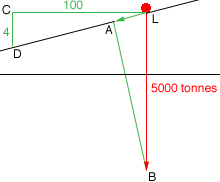Quandaries and Queries I've got a maths problem to solve but have no idea how to tackle it's prob very simple i just can't see it, i've changed the data in this example so i know how to do it for the real question hear goes! A 50 tonne lorry is parked on a slope with a gradient of 4%. Due to the self weight of the lorry, what is the force * acting down the slope * acting normal (at right angles) to the slope   any help would be greatfully received thanks Hi Aaron, I drew a vector diagram. L is the lorry and the vector LB represents the force of the 500 tonne lorry acting vertically.I then drew a perpendicular from B to the slope to intersect the slope at A. The vector LA is then the component of LB in the direction of the slope and hence a vector the represents the force acting down the slope. To find the length of LA I drew a horizontal line from L to a point C and then dropped a vertical line from B to intersect the slope at D. Since the slope has a 4% grade the ratio of |CD| to |LC| is 4 to 100. From the diagram angles DLC and LBA are equal and thus the right triangles DLC and LBA are similar. Use the Theorem of Pythagoras to find |LD|. Now you can use the similarity of the triangles to find the length of LA which is the magnitude of the force acting down the slope. Can you now find the magnitude of the component of the vector LB acting perpendicular to the slope? Penny Go to Math Central